# AP Calculus AB Practice Test 3

### Test Information10 questions20 minutes

Calculator Disallowed

1. If f(x) = 53x, then f′(x) =

2. A solid is generated when the region in the first quadrant enclosed by the graph of y = (x2 + 1)3, the line x = 1, the x-axis, and the y-axis is revolved about the x-axis. Its volume is found by evaluating which of the following integrals?

3.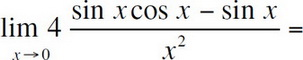4. If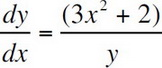and y = 4 when x = 2, then when x = 3, y =

5.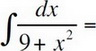6. If f(x) = cos3 (x + 1), then f′(π) =

7.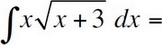8. If f(x) = ln(ln(1 -x)), then f′(x) =

9.10.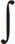tab6x sec2xdx =